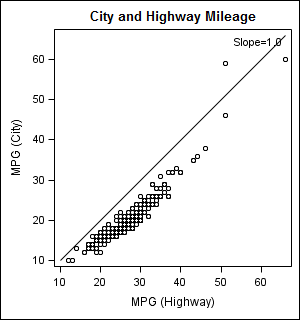PGStats
Opal | Level 21

## Axes aspect ratio of 1:1

Hello, is there a way, other than trial and error, to get the scale of SAS-GRAPH or SG- graphics X and Y axes to be equal?

PG

PG
10 REPLIES 10

## Re: Axes aspect ratio of 1:1

In SAS Graph the AXIS statment has the option

LENGTH=axis length <units

specifies the length of the axis in number of units. If you request a length that cannot fit the display, a warning message is written to the log and your graph may produce unexpected results. (from the online help)

## Re: Axes aspect ratio of 1:1

For example ...

In the following graphs, I use:

axis1 length=2.3in offset=(0,0) order=(0 to 60 by 10) minor=none;

axis2 length=2.3in offset=(0,0) order=(0 to 60 by 10) minor=none;

And then in the gplot I use them as ...

vaxis=axis1 haxis=axis2

Which produces these nice plots with proportional axes:

for the full code, see...PGStats
Opal | Level 21

## Re: Axes aspect ratio of 1:1

Thanks!

Very nice graphs! But as usual, a lot of tinkering required. One has to figure out the X and Y ranges, decide on tick mark values and translate that into physical dimensions. Quite a bit more trouble than I got used to expect since the advent of SG- procedures... and incompatible with BY processing unless one wants UNIFORM graphs.

I don't know what the demand would be for a feature such as XYRATIO=, but I am sure any improvement in that direction would promote the proper visual representation of related units.

PG

PG

## Re: Axes aspect ratio of 1:1

I put in a request for this enhancement a while back - I'll add a note to that request to let developers know you're also interested.

S0307966 NewFeature:GPLOT SUGGESTION:

ADD OPTION TO PRESERVE 1/1 ASPECT RATIO OF THE AXESPGStats
Opal | Level 21

## Re: Axes aspect ratio of 1:1

Great! Thank you Robert. GPLOT is only one place where this would be useful, GCONTOUR when used in conjunction with geotatistics procedures, for example, could also benefit from that option. - PG

PGJay54
Meteorite | Level 14

## Re: Axes aspect ratio of 1:1

With SAS 9.2 you can use GTL with LAYOUT OVERLAYEQUATED to create a graph with a real 1:1 ratio.  Here is an example of the usage of such a layout with a SCATTERPLOT statement:  In this case the LINEPARM with slope=1 is actually a 45 degree line in the graph.

proc template;
define statgraph equated;
begingraph;
entrytitle 'City and Highway Mileage';
layout overlayequated / equatetype=square;
scatterplot x=mpg_highway y=mpg_city;
lineparm x=10 y=10 slope=1 / curvelabel='Slope=1.0';
endlayout;
endgraph;
end;
run;

ods graphics / reset width=3in height=3.2in imagename='Equated';
proc sgrender data=sashelp.cars template=equated;
run;

Here is another example of usage of the Equated layout:  Map Overlays.  LAYOUT OVERLAYEQUATED has three different EquateType of FIT, SQUARE and EQUATE.  You can make the scales equal, but there not a way to have guaranteed equal lengths.PGStats
Opal | Level 21

## Re: Axes aspect ratio of 1:1

Thank you Sanjay! Which of GPLOT or SGPLOT will offer this as a simple option first?PG

PG

## Re: Axes aspect ratio of 1:1

My vote is on SGPLOT because I've been advocating for this for a while and I thik they're getting tired of hearing me complain.

RickJay54
Meteorite | Level 14

## Re: Axes aspect ratio of 1:1

It will be in the SG family, but it is not as simple as adding to SGPLOT.  We need to consider if this should really be a separate procedure, since many of the options (and statements) avaialble in SGPLOT will not be valid for this case.

We are considering a SGEQUATED procedure.  This will only plots with allow numeric (linear) values on the axes, and many axis options will be common (to X & Y), and will need a EquatedAxis statement.  There are benefits to separating it out (cleaner syntax and implementation).  I don't yet see the benefit of adding this into SGPLOT.

Do you see any benefits of adding this feature into the SGPLOTprocedure?  In GTL, this is a whole separate layout container.  Opinions?PGStats
Opal | Level 21

## Re: Axes aspect ratio of 1:1

From my casual user perspective, this feature would simply affect the scaling of the axes, and only when they are both continuous and of the same type (linear, log or time). I don't see what else would be affected. I enjoy very much the (apperent) simplicity of statistical graphics procedures. I trust you guys to keep them that way, as much as possible. Thanks!

PG

PG
Discussion stats
• 10 replies
• 2373 views
• 6 likes
• 5 in conversation